International
Tables for
Crystallography
Volume F
Crystallography of biological macromolecules
Edited by M. G. Rossmann and E. Arnold

International Tables for Crystallography (2006). Vol. F, ch. 11.4, p. 233   | 1 | 2 |

Section 11.4.8. Multiplicative corrections (scaling)

Z. Otwinowskia* and W. Minorb

aUT Southwestern Medical Center at Dallas, 5323 Harry Hines Boulevard, Dallas, TX 75390-9038, USA, and bDepartment of Molecular Physiology and Biological Physics, University of Virginia, 1300 Jefferson Park Avenue, Charlottesville, VA 22908, USA
Correspondence e-mail:  zbyszek@mix.swmed.edu

11.4.8. Multiplicative corrections (scaling)

| top | pdf |

Proper error estimation requires the use of Bayesian reasoning and a multi-component error model (Schwarzenbach et al., 1989; Evans, 1993). In SCALEPACK, the estimated error of the measurement is enlarged by a fraction of the expected, rather than the observed, intensity. This algorithm reduces the bias towards reflections with an integrated intensity below the average.

The scaling model allows for a large number of diverse components to contribute to the multiplicative correction factor s for each observation,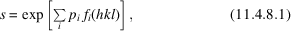whereare a priori unknown coefficients of the scaling components andrepresent different functional dependence of the scale factor for each observation. The simplest scaling model has a separate scale factor for each group (batch) of data, for example, one scale factor per image. In such a case,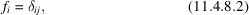where j is the batch index for a particular reflection. For resolution-dependent decay, represented by one temperature factor per batch of data,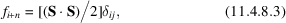where n is the number of batches needed to makerepresent the logarithm of the overall ith batch scale factor and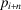represents the temperature factor of batch i. S is the scattering vector for each reflection.

Coefficients of crystal absorption are much more complex. Scaling coefficients are associated with spherical harmonics (Katayama, 1986; Blessing, 1995) as a function of the direction of vector S, expressed in the coordinate system of the rotating crystal. Each spherical harmonic index lm has two (or, in the case of, one)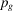coefficients. One of these spherical harmonic functions is given by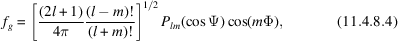where Ψ and Φ are polar-coordinate angles of vector S in the crystal coordinate system, and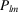is a Legendre polynomial (Press et al., 1989). The other spherical harmonic of index lm has a sine instead of a cosine as the last term.

The multiplicative factor is applied to each observation and its σ to obtain the corrected intensity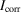and associated σ. The averaged intensity over all symmetry-related reflections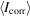is obtained by solving the two following equations: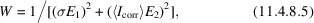whereandare the user-specified error scale factor and estimated error, respectively, and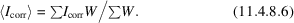Thus,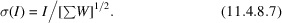During parameter refinement, the scale (and B, if requested) for all scaled batches are refined against the difference between the's and's for individual measurements, summed over all reflections (Fox & Holmes, 1966; Arndt & Wonacott, 1977; Stuart & Walker, 1979; Leslie & Tsukihara, 1980; Rossmann & Erickson, 1983; Walker & Stuart, 1983; Rossmann, 1984; Schutt & Evans, 1985; Stuart, 1987; Takusagawa, 1987; Tanaka et al., 1990).'s are recalculated in each cycle of refinement. There is full flexibility in the treatment of anomalous pairs. They can be assumed to be equivalent (or not) and they may be merged (or not). This approach allows the crystallographer to choose the best scaling and merging strategy.

11.4.8.1. Polarization

| top | pdf |

A polarization correction may be applied during DENZO calculations. Sometimes the exact value of polarization is not known. This error may be corrected during the scaling procedure. This feature can be used to refine the polarization at synchrotron beamlines. Very high resolution data should be used for this purpose.

References

Arndt, U. W. & Wonacott, A. J. (1977). The rotation method in crystallography. Amsterdam: North Holland.Google Scholar
Blessing, R. H. (1995). An empirical correction for absorption anisotropy. Acta Cryst. A51, 33–38.Google Scholar
Evans, P. (1993). Data reduction: data collection and processing. In Proceedings of the CCP4 study weekend. Data collection and processing, 29–30 January, edited by L. Sawyer, N. Isaac & S. Bailey, pp. 114–123. Warrington: Daresbury Laboratory.Google Scholar
Fox, G. C. & Holmes, K. C. (1966). An alternative method of solving the layer scaling equations of Hamilton, Rollett and Sparks. Acta Cryst. 20, 886–891.Google Scholar
Katayama, C. (1986). An analytical function for absorption correction. Acta Cryst. A42, 19–23.Google Scholar
Leslie, A. G. W. & Tsukihara, T. (1980). A strategy for collecting isomorphous derivative data with the oscillation method. J. Appl. Cryst. 13, 304–305.Google Scholar
Press, W. H., Flannery, B. P., Teukolsky, S. A. & Vetterling, W. T. (1989). Numerical recipes – the art of scientific computing. Cambridge University Press.Google Scholar
Rossmann, M. G. (1984). Synchrotron radiation studies of large proteins and supramolecular structures. In Proceedings of the study weekend at Daresbury Laboratory. Biological systems: structure and analysis, 24–25 March, edited by G. P. Diakun & C. D. Garner, pp. 28–40. Daresbury: Science and Engineering Research Council.Google Scholar
Rossmann, M. G. & Erickson, J. W. (1983). Oscillation photography of radiation-sensitive crystals using a synchrotron source. J. Appl. Cryst. 16, 629–636.Google Scholar
Schutt, C. E. & Evans, P. R. (1985). Relative absorption correction for rotation film data. Acta Cryst. A41, 568–570.Google Scholar
Schwarzenbach, D., Abrahams, S. C., Flack, H. D., Gonschorek, W., Hahn, Th., Huml, K., Marsh, R. E., Prince, E., Robertson, B. E., Rollett, J. S. & Wilson, A. J. C. (1989). Statistical descriptors in crystallography. Acta Cryst. A45, 63–75.Google Scholar
Stuart, D. (1987). Absorption correction. In Proceedings of the Daresbury study weekend at Daresbury Laboratory, 23–24 January, edited by J. R. Helliwell, P. A. Machin & M. Z. Papiz, pp. 25–38. Warrington: Daresbury Laboratory.Google Scholar
Stuart, D. & Walker, N. (1979). An empirical method for correcting rotation-camera data for absorption and decay effects. Acta Cryst. A35, 925–933.Google Scholar
Takusagawa, F. (1987). A simple method of absorption and decay correction in intensities measured by area-detector X-ray diffractometer. J. Appl. Cryst. 20, 243–245.Google Scholar
Tanaka, I., Yao, M., Suzuki, M., Hikichi, K., Matsumoto, T., Kozasa, M. & Katayama, C. (1990). An automatic diffraction data collection system with an imaging plate. J. Appl. Cryst. 23, 334–339.Google Scholar
Walker, N. & Stuart, D. (1983). An empirical method for correcting diffractometer data for absorption effects. Acta Cryst. A35, 158–166.Google Scholar